# Solution of Differential Equation for a Tank Problem

A tank contains 2,500 liters of water in which 5 kg of salt is dissolved at the initial time. The solution drains from the tank at a rate of 100 l/min and another water solution (with 10 g of salt per 1 l of water) enters the tank at the same rate. Assume that the solution is well stirred (the salt concentration is the same everywhere in the tank). What is the equation for finding the amount of salt at any time? Draw the plot. Determine the time when there will be 20 kg of salt in the tank.

Solution

Let’s visualize the scheme for better understanding: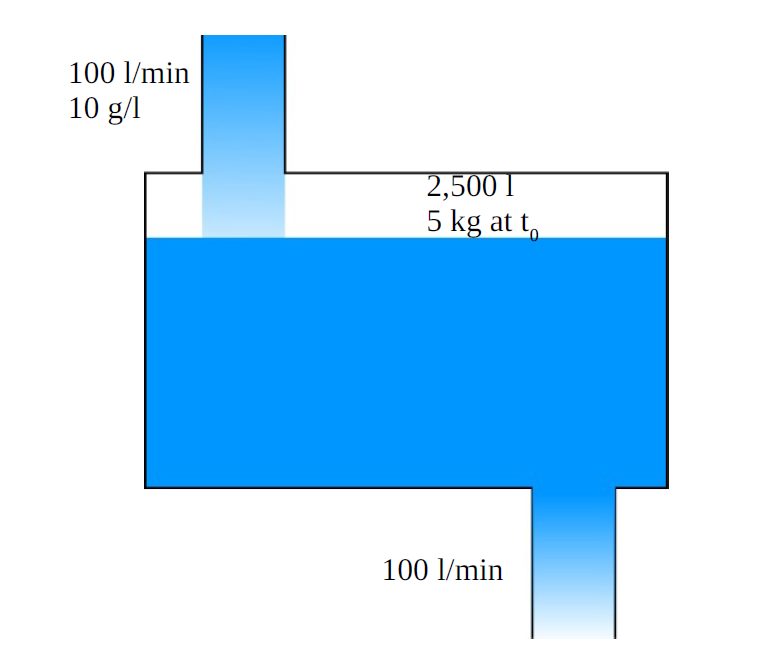The quantity of the salt in the tank at any time is the difference between the rate the salt flows in and the rate the salt flows out. The concentration of the salt in the solution that flows out is the same as in the tank itself: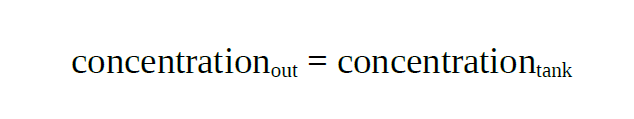The rate of the salt that flows in can be found as the product of the concentration and the rate of the solution: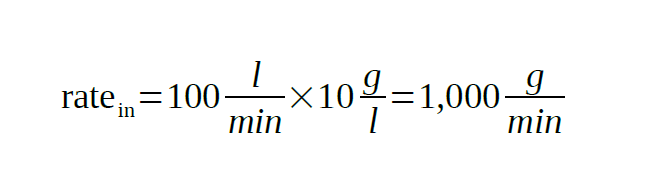The rate of the salt flowing out is: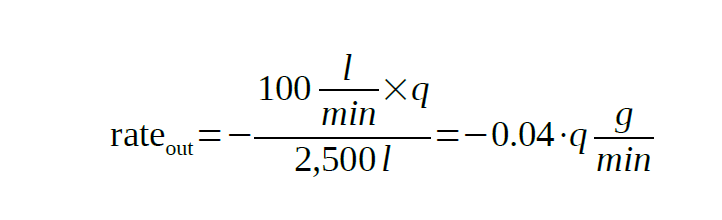Where q is the quantity of the salt in the tank.

Thus, the rate of the salt in the tank is: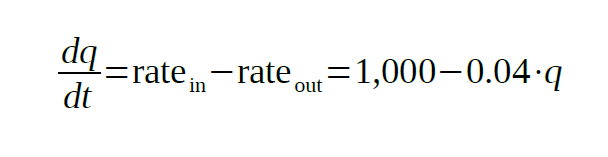To solve the above equation, we need to rewrite it: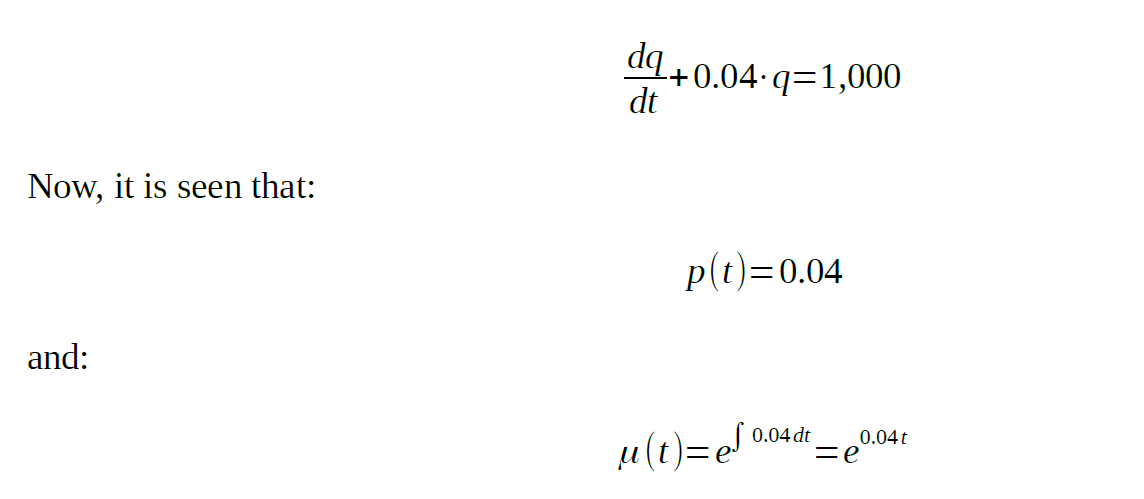Multiplying the above value with the rewritten equation: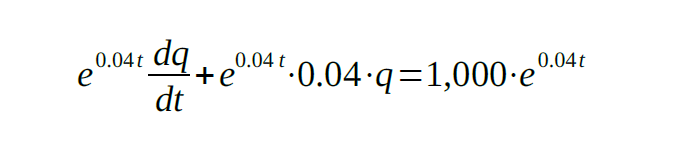Simplifying: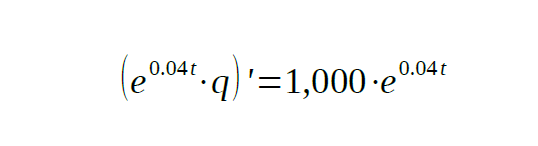Integrating the expression: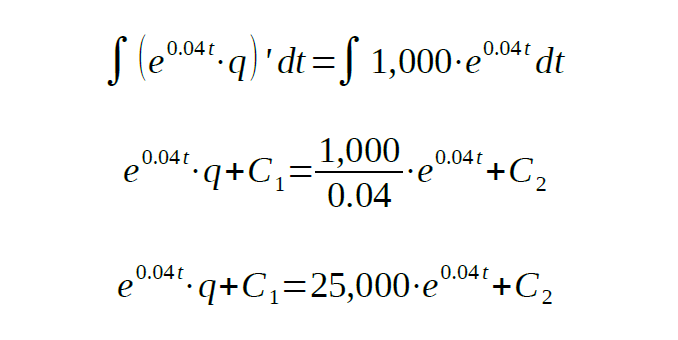As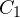and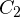are unknown, we can rewrite: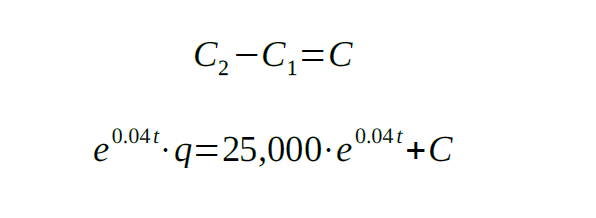At last, the general solution is: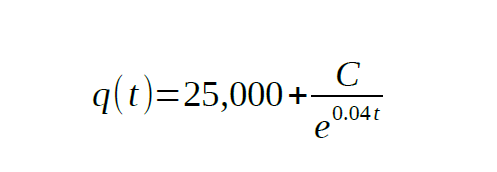The initial condition is: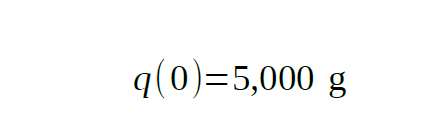So, we can find the unknown constant C: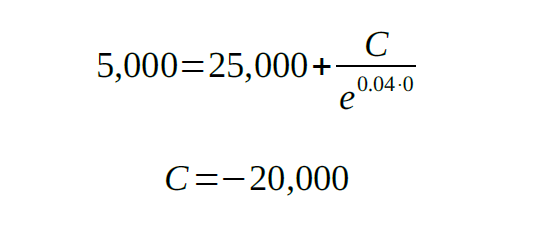The final solution is: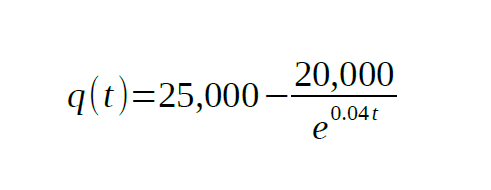The plot is shown below: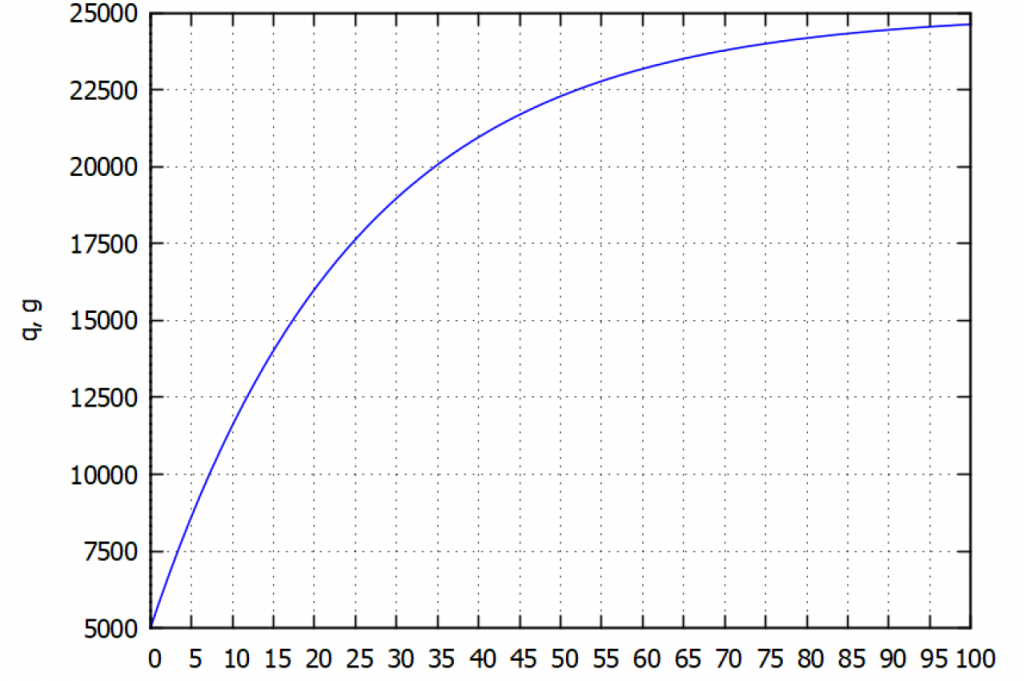To determine the time when there will be 20 kg of salt dissolved, we need to solve the above equation for t: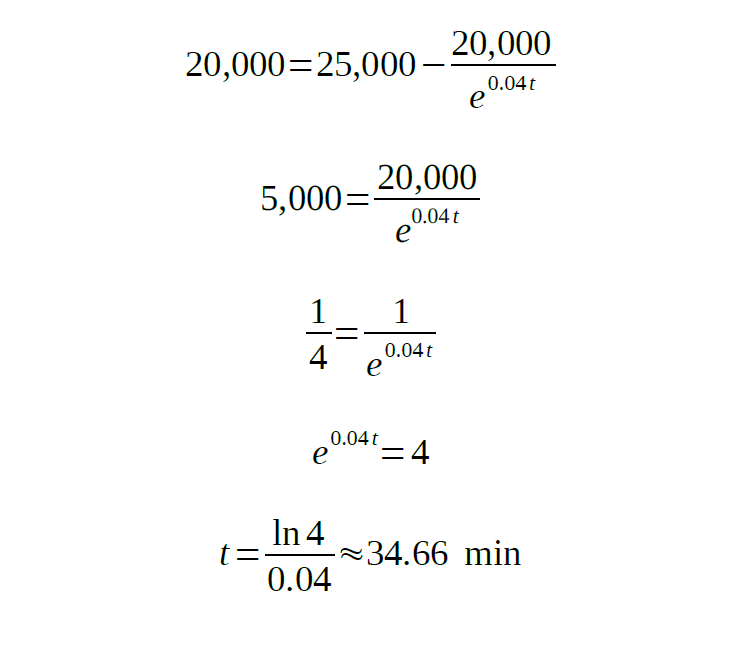Mathematics isn’t the easiest discipline. Sooner or later you’ll have to deal with it, whether at school or college. Maybe you’ll also have to help your kids with various mathematical assignments. it might be hard, especially for people who are used to dealing with words, not numbers.

AssignmentShark is a service that helps people to deal with technical and calculus homework: statistics, numerical analysis, IT, engineering, etc. The presented example was provided by our expert. If you have to deal with a differential equation for the tank problem, read our article thoroughly. In such a way, you’ll get a better understanding of this assignment.

You may also check other posts on our blog along with the presented salt tank problem differential equation. If you need assistance with your technical homework, don’t hesitate to apply to our service!